# Layers¶

In this section, we will cover all relevant layers implemented by Slugnet, and their specific use cases. This includes convolutional deep networks and layers associated with them.

## Fully Connected Deep Networks¶

Slugnet implements fully connected deep networks via the Dense layer. When operating in feedforward mode, the dense layer computes the following termwhereis activated output,is the activation function,are weights,is the bias term. The dense layer does not implement any activation function, instead it is injected at runtime via the activation parameter. This mean that on feedforward, the dense layer is incredibly simple, it performs matrix multiplication between an input matrix and a matrix of weights, then adds a bias vector, and that’s it.

On feed backward, or backpropagation, the dense layer is responsible for calculating two values. The value definedwill be used to calculate the weight and bias gradient at this layer. The valuewill be used to calculate gradients at all previous layers. This process is easy to follow in the backpropagation algorithm given in the introduction section of this documentation.When looking at the implementation of Dense, there is a notable absence ofand. This is because their dot product is calculated in the previous layer. The model propagates that gradient to the current layer.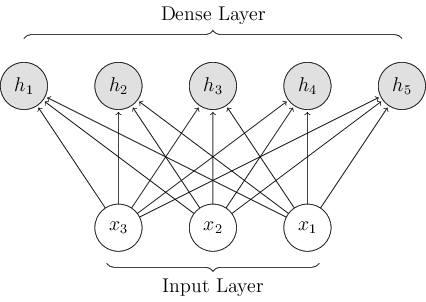Figure 1: A depiction of a five unit dense layer. The dense layer is connected to a three unit input layer. The arrows going from the input layer to the dense layer represent weights that are multuplied by the values given by the input layer. The resulting values are represented by the gray nodes in the hidden dense layer.

class slugnet.layers.Dense(outshape, inshape=None, activation=<slugnet.activation.Noop object>, init=<slugnet.initializations.GlorotUniform object>)[source]

Bases: slugnet.layers.Layer

A common densely connected neural network layer.

Parameters: outshape (int) – The output shape at this layer. inshape (int) – The input shape at this layer. activation (slugnet.activation.Activation) – The activation function to be used at the layer. init (slugnet.initializations.Initializer) – The initialization function to be used
backprop(nxt_grad)[source]

Computes the derivative for the next layer and computes update for this layers weights

An example of using two dense layers to train a multi-layer deep network to classify mnist data can be seen below.

from slugnet.activation import ReLU, Softmax
from slugnet.layers import Dense
from slugnet.loss import SoftmaxCategoricalCrossEntropy as SCCE
from slugnet.model import Model
from slugnet.optimizers import RMSProp
from slugnet.data.mnist import get_mnist

X, y = get_mnist()
model = Model(lr=0.01, n_epoch=3, loss=SCCE(),
metrics=['loss', 'accuracy'],
optimizer=RMSProp())

model.fit(X, y)


If you have slugnet installed locally, this script can be executed by running the following command. It will output training and validation statistics to stdout as the model is trained.



## Convolutional Deep Networks¶

Convolutional deep networks are most often used in image classification tasks. There are several specialized layers used in these networks. The most obvious is the convolution layer, less obvious are pooling layers, specifically max-pooling and mean-pooling. In this section we will mathematically review all these layers in depth.

### Convolution Layer¶

In the general case, a discrete convolution operation implements the function:whereis the input andis the kernel, or in some cases the weighting function.

In the case of convolutional deep networks, the input is typically a two dimensional image, and it follows that we have a two dimensional kernel. Now we can write out convolution function with both axes:Note that we can write the infinite sum over the domains ofandas discrete sums because we assume that the kernelis zero everywhere but the set of points in which we store data .

The motivation for using the convolution operation in a deep network is best described using an example of an image. In a densely connected deep network, each node at layeris connected to every node at layer. This does not lend itself to image processing, where location of a shape relative to another shape is important. For instance, finding a right angle involves detecting two edges that are perpendicular, and whose lines cross one another. If we make the nonzero parts of the kernel smaller than the input image, we can process parts of the image at a time, thereby ensuring locality of the input signals. To process the entire image, we slide the kernel over the input, along both axes. At each step, an output is produced which will be used as input for the next layer. This configuration allows us to learn the parameters of the kernelthe same way we’d learn ordinary parameters in a densely connected deep network.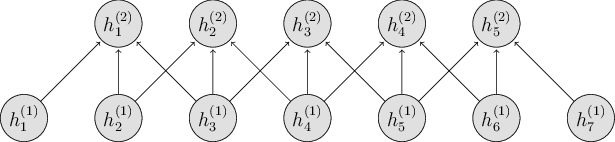Figure 3: Note how the convolution layer connects nodes that are close to one another. This closeness is determined by the size of the kernel. In this case we have an input in, a kernel in, and an output in.

From figure 3, we can see that the output size can be determined from the input size and kernel size. The equation is given byFigure 3 features a one dimensional input and output. As we mentioned earlier, most convolutional deep networks feature two dimensional inputs and outputs, such as images. In figure 4, we show how the convolution operation behaves when we are using two dimensional inputs, kernels, and outputs.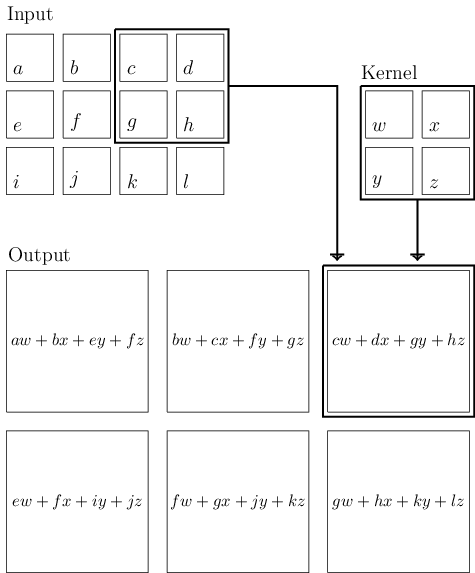Figure 4: An example of a two dimension convolution operation. The input is an image in, and the kernel is in. As the kernel is slid over the input with a stride width of one, an output inis produced. In the example, the arrows and boxes demonstrate how the upper-right portion of the input image are compbined with the kernel parameters to produce the upper right unit of output.

–Modified from source: Goodfellow, Bengio, Courville (Deep Learning, 2016, Figure 9.1).

The stride width determines how far the kernel moves at each step. Of course, to learn anything interesting, we require multiple kernels at each layer. These are all configurable hyperparameters that can be set upon network instantiation. When the network is operating in feedforward mode, the output at each layer is a three dimensional tensor, rather than a matrix. This is due to the fact that each kernel produces its own two dimensional output, and there are multiple kernels at every layer.

class slugnet.layers.Convolution(nb_kernel, kernel_size, stride=1, inshape=None, init=<slugnet.initializations.GlorotUniform object>, activation=None)[source]

Bases: slugnet.layers.Layer

A layer that implements the convolution operation.

Parameters: nb_kernel (int) – The number of kernels to use. kernel_size ((int, int)) – The size of the kernel as a tuple, heigh by width stride (int) – The stide width to use init (slugnet.initializations.Initializer) – The initializer to use activation (slugnet.activation.Activation) – The activation function to be used at the layer.

### Pooling¶

Mean pooling is a method of downsampling typically used in convolutional deep networks. Pooling makes the representations at a subsequent layer approximately invariant to translations of the output from the previous layer . This is useful when we care about the presence of some feature but not necessarily the exact location of the feature within the input.

The mean pooling operation implements the functionwhereare vectors of kernel indices over the image. This operation is depicted in figure 2.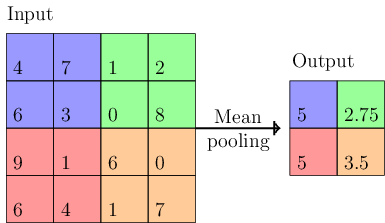Figure 5: A visual representation of the mean pooling operation. Color coded patches are combined via arithmetic average and included in an output matrix.

The max-pooling operation implements the functionwhereare vectors of kernel indices over the image. This operation is depicted in figure 3.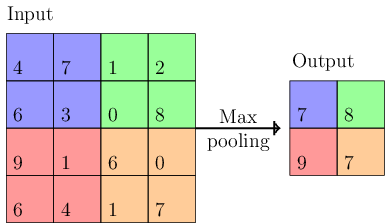Figure 6: A visual representation of the max pooling operation. Color coded patches are downsampled by taking the maximum value found in the patch.

class slugnet.layers.MeanPooling(pool_size, inshape=None)[source]

Bases: slugnet.layers.Layer

Pool outputs using the arithmetic mean.

We have now documented all the necessary parts of a convolutional deep network. This makes training one to classify mnist data simple.

import numpy as np

from slugnet.activation import ReLU, Softmax
from slugnet.layers import Convolution, Dense, MeanPooling, Flatten
from slugnet.loss import SoftmaxCategoricalCrossEntropy as SCCE
from slugnet.model import Model
from slugnet.optimizers import SGD
from slugnet.data.mnist import get_mnist

X, y = get_mnist()
X = X.reshape((-1, 1, 28, 28)) / 255.0
np.random.seed(100)
X = np.random.permutation(X)[:1000]
np.random.seed(100)
y = np.random.permutation(y)[:1000]

model = Model(lr=0.001, n_epoch=100, batch_size=3, loss=SCCE(),
metrics=['loss', 'accuracy'], optimizer=SGD())

model.add_layer(Convolution(1, (3, 3), inshape=(None, 1, 28, 28)))